Question

# Let X have a normal distribution with mean μ and variance σ ^2 . The highest...

Let X have a normal distribution with mean μ and variance σ ^2 . The highest value of the pdf is equal to 0.1 and when the value of X is equal to 10, the pdf is equal to 0.05. What are the values of μ and σ?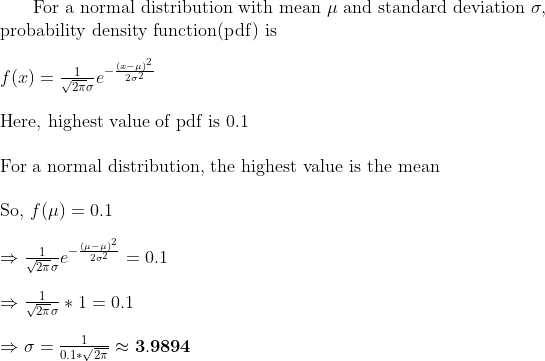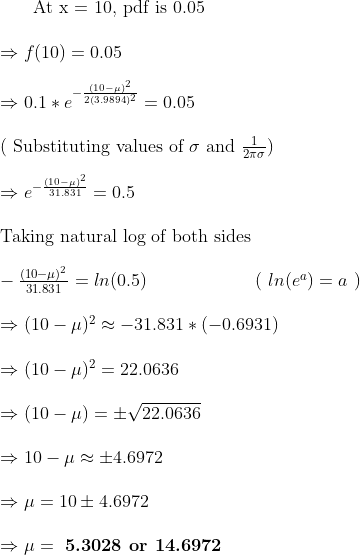#### Earn Coins

Coins can be redeemed for fabulous gifts.

Similar Homework Help Questions
• ### Let fy(x, μ, σ) stand for the probability distribution function (PDF) for the normal distribution with...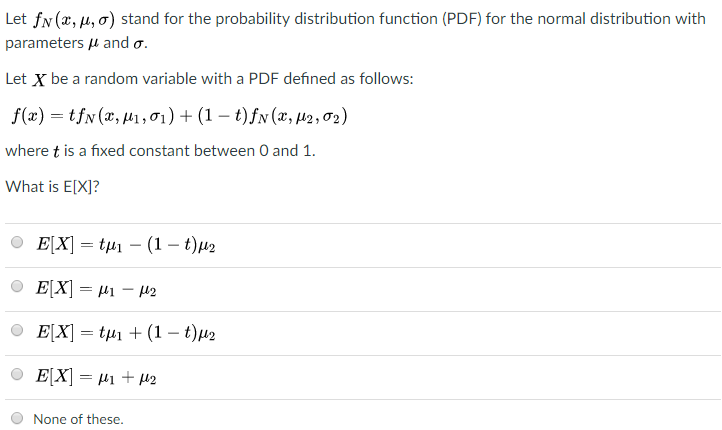Let fy(x, μ, σ) stand for the probability distribution function (PDF) for the normal distribution with parameters μ and σ. Let X be a random variable with a PDF defined as follows: where t is a fixed constant between O and 1. What is E[XI? None of these

• ### Let X be normal with mean μ and standard deviation σ. a) The cumulative distribution satisfies...

Let X be normal with mean μ and standard deviation σ. a) The cumulative distribution satisfies F(σ) = 50% b) X is bimodal with modes as μ- σ and μ+σ c) F(μ-σ) = 1-F(μ+σ) d) Z = (X-μ)/σ is the standard unit normal. e) If a<c<b, the (F(b)-F(a))>(F(c)-F(a))

• ### Problem3 (15 points (a) (8 points) Let x, X, be a random sample from normal distribution NG, σ, ....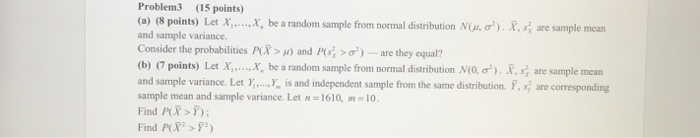Problem3 (15 points (a) (8 points) Let x, X, be a random sample from normal distribution NG, σ, . s are sample mean and sample variance. Consider the probabilities PC, μ) and PS? σ)-are they equal? (b) (7 points) Let X, , ,X, be a random sample from normal distribution Mo, σ, R, s are sample mean and sample variance. Let y.... is and independent sample from the same distribution. Y, s are corresponding sample mean and sample variance. Let...

• ### 12 Find 10. Let X be a Gaussian rv with mean μ and variance σ, or...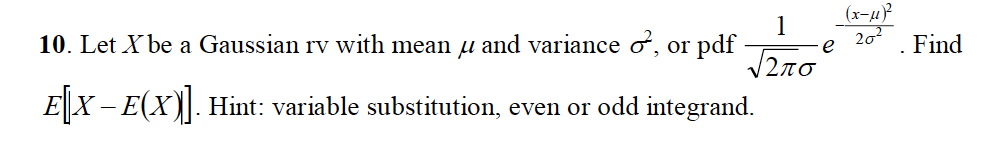12 Find 10. Let X be a Gaussian rv with mean μ and variance σ, or pdf-l-e 2ơ . Find E X-E(Xt]. Hint: variable substitution, even or odd integrand.

• ### 8) Let Yi, X, denote a random sample from a normal distribution with mean μ and...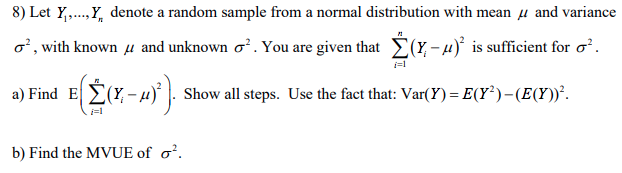8) Let Yi, X, denote a random sample from a normal distribution with mean μ and variance σ , with known μ and unknown σ' . You are given that Σ(X-μ)2 is sufficient for σ a) Find El Σ(X-μ). |. Show all steps. Use the fact that: Var(Y)-E(P)-(BY)' i-1 b) Find the MVUE of σ.

• ### 2. Suppose Yi,.. narei normal random variables with normal distribution with unknown mean and variance, μ...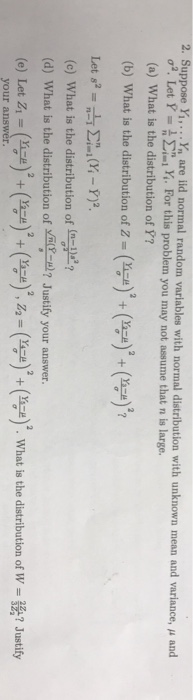2. Suppose Yi,.. narei normal random variables with normal distribution with unknown mean and variance, μ and or. Let Y-욤 Σ;..x. For this problem, you may not assume that n is large. (a) What is the distribution of Y? (b) what is the distribution of z-(yo), (en, (n-) (c) what is the distribution of (n-p? (d) What is the distribution of Justify your answer. (e) Let Zi-(ga)' + (-)' + (yo)", z2 = (속)' + (n-e)' what is the distribution...

• ### Let X1,X2, , Xn be a random sample from a normal distribution with a known mean μ (xi-A)2 and variance σ unknown. Let ơ-- Show that a (1-α) 100% confidence interval for σ2 is (nơ2/X2/2,n, nơ2A-a/2,n)...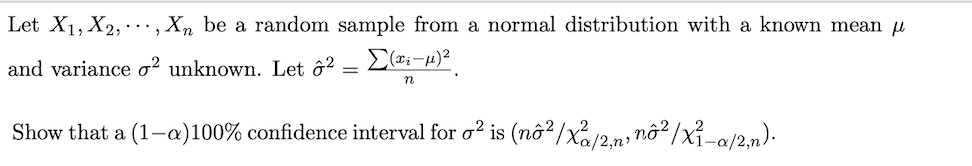Let X1,X2, , Xn be a random sample from a normal distribution with a known mean μ (xi-A)2 and variance σ unknown. Let ơ-- Show that a (1-α) 100% confidence interval for σ2 is (nơ2/X2/2,n, nơ2A-a/2,n). Let X1,X2, , Xn be a random sample from a normal distribution with a known mean μ (xi-A)2 and variance σ unknown. Let ơ-- Show that a (1-α) 100% confidence interval for σ2 is (nơ2/X2/2,n, nơ2A-a/2,n).

• ### Let the random variable X follow a normal distribution with μ =40 and σ^2 =81. The...

Let the random variable X follow a normal distribution with μ =40 and σ^2 =81. The probability is 0.03 that X is in the symmetric interval about the mean between which two numbers? Round to one decimal place as needed. Use ascending​ order

• ### Let Xi, x,, ,X, be independent random variables with mean and variance σ . Let Y1-Y2, , Y, be independent random variables with mhean μ and variance a) Compute the expected value of W b) For what val...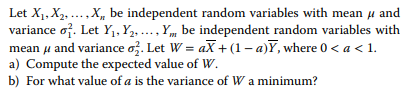Let Xi, x,, ,X, be independent random variables with mean and variance σ . Let Y1-Y2, , Y, be independent random variables with mhean μ and variance a) Compute the expected value of W b) For what value of a is the variance of W a minimum? σ: Let W-aX + (1-a) Y, where 0 < a < 1. Let Xi, x,, ,X, be independent random variables with mean and variance σ . Let Y1-Y2, , Y, be independent random...

• ### For a Normal distribution with mean, μ=2, and standard deviation, σ=4, 30% of all observations have...

For a Normal distribution with mean, μ=2, and standard deviation, σ=4, 30% of all observations have a value less than Round to 4 decimal places.